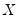User’s Guide : Basic Single Equation Analysis : Basic Regression Analysis : Estimation Problems

Estimation Problems
Exact Collinearity
If the regressors are very highly collinear, EViews may encounter difficulty in computing the regression estimates. In such cases, EViews will issue an error message “Near singular matrix.” When you get this error message, you should check to see whether the regressors are exactly collinear. The regressors are exactly collinear if one regressor can be written as a linear combination of the other regressors. Under exact collinearity, the regressor matrixdoes not have full column rank and the OLS estimator cannot be computed.
You should watch out for exact collinearity when you are using dummy variables in your regression. A set of mutually exclusive dummy variables and the constant term are exactly collinear. For example, suppose you have quarterly data and you try to run a regression with the specification:
y c x @seas(1) @seas(2) @seas(3) @seas(4)
EViews will return a “Near singular matrix” error message since the constant and the four quarterly dummy variables are exactly collinear through the relation:
c = @seas(1) + @seas(2) + @seas(3) + @seas(4)
In this case, simply drop either the constant term or one of the dummy variables.
The textbooks listed above provide extensive discussion of the issue of collinearity.